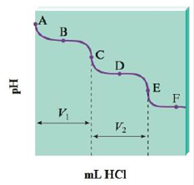# The titration of Na 2 CO 3 with HCl bas the following qualitative profile: a. Identify the major species in solution at points A–F. b. Calculate the pH at the halfway points to equivalence, B and D. ( Hint: Refer to Exercise 113.)### Chemistry: An Atoms First Approach

2nd Edition
Steven S. Zumdahl + 1 other
Publisher: Cengage Learning
ISBN: 9781305079243

#### Solutions

Chapter
Section### Chemistry: An Atoms First Approach

2nd Edition
Steven S. Zumdahl + 1 other
Publisher: Cengage Learning
ISBN: 9781305079243
Chapter 14, Problem 115CP
Textbook Problem
100 views

## The titration of Na2CO3 with HCl bas the following qualitative profile:a. Identify the major species in solution at points A–F.b. Calculate the pH at the halfway points to equivalence, B and D. (Hint: Refer to Exercise 113.)

(a)

Interpretation Introduction

Interpretation: The titration curve of Na2CO3 and HCl is given. Various questions based on the given curve are to be answered.

Concept introduction: Titration is a quantitative chemical analysis method that is used for the determination of concentration of an unknown solution. In acid base titration, the neutralization of either acid or base is done with a base or acid respectively of known concentration. This helps to determine the unknown concentration of acid or base.

When the amount of the titrant added is just sufficient for the neutralization of analyte is called equivalence point. At this point equal equivalents of both the acid and base are added.

### Explanation of Solution

Explanation

There is presence of Na+ in all the solutions. There occurs formation of HCO3 after the HCl has reacted with CO32 . This is shown by points A-C. Once all the CO32 has reacted, the proton from acid reacts with the other available base, that is HCO3 . It is shown by the points C-E. The point C is the first equivalence point and point E is the second equivalence point. The species that are shown by different points are given as,

 Point A CO32− , Na+ and H2O Point B CO32− , HCO3− , Na+ , Cl− , H2

(b)

Interpretation Introduction

Interpretation: The titration curve of Na2CO3 and HCl is given. Various questions based on the given curve are to be answered.

Concept introduction: Titration is a quantitative chemical analysis method that is used for the determination of concentration of an unknown solution. In acid base titration, the neutralization of either acid or base is done with a base or acid respectively of known concentration. This helps to determine the unknown concentration of acid or base.

When the amount of the titrant added is just sufficient for the neutralization of analyte is called equivalence point. At this point equal equivalents of both the acid and base are added.

To determine: The value of pH at the halfway points to the equivalence points, B and D.

### Still sussing out bartleby?

Check out a sample textbook solution.

See a sample solution

#### The Solution to Your Study Problems

Bartleby provides explanations to thousands of textbook problems written by our experts, many with advanced degrees!

Get Started

Find more solutions based on key concepts
Divide into two groups. One group will argue in favor of the use of ergogenic aids by athletes, and one group w...

Nutrition: Concepts and Controversies - Standalone book (MindTap Course List)

What factors influence the breaking of a wind wave?

Oceanography: An Invitation To Marine Science, Loose-leaf Versin

Two long, straight wires cross each other perpendicularly as shown in Figure P30.63. The wires are thin so that...

Physics for Scientists and Engineers, Technology Update (No access codes included)